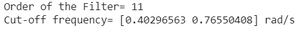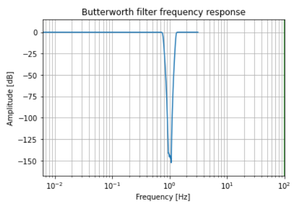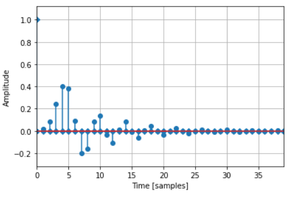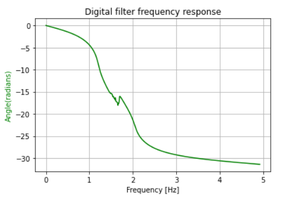Related Articles
Digital Band Reject Butterworth Filter in Python
• Last Updated : 03 Jan, 2021

In this article, we are going to discuss how to design a Digital Band Reject Butterworth Filter using Python. The Butterworth filter is a type of signal processing filter designed to have a frequency response as flat as possible in the pass band. Let us take the below specifications to design the filter and observe the Magnitude, Phase & Impulse Response of the Digital Butterworth Filter.

## What is Digital Bandreject Filter?

A band-pass filter is a filter that passes frequencies within a range and rejects frequencies outside that range.

## How it’s different from Highpass, Lowpass & Bandpass:

The main difference can be spotted by observing the magnitude response of the Band Pass Filter. In the Band-Reject filter, all the signals between the specified frequency range get rejected by the filter.

The specifications are as follows:

• The sampling rate of 12 kHz.
• Pass band edge frequencies are 2100 Hz & 4500 Hz.
• Stop band edge frequencies are 2700 Hz & 3900 Hz.
• Pass band ripple of 0.6 dB.
• Minimum stop band attenuation of 45 dB.

We will plot the magnitude, phase, and impulse response of the filter.

### Step-by-step Approach:

Before starting, first, we will create a user-defined function to convert the edge frequencies, we are defining it as the convert() method.

## Python3

 `# explicit function to convert``# edge frequencies`` ` ` ` `def` `convertX(f_sample, f):``    ``w ``=` `[]`` ` `    ``for` `i ``in` `range``(``len``(f)):``        ``b ``=` `2``*``((f[i]``/``2``) ``/` `(f_sample``/``2``))``        ``w.append(b)`` ` `    ``omega_mine ``=` `[]`` ` `    ``for` `i ``in` `range``(``len``(w)):``        ``c ``=` `(``2``/``Td)``*``np.tan(w[i]``/``2``)``        ``omega_mine.append(c)`` ` `    ``return` `omega_mine`

Step 1: Importing all the necessary libraries.

## Python3

 `# import required modules``import` `numpy as np``import` `matplotlib.pyplot as plt``from` `scipy ``import` `signal``import` `math`

Step 2: Define variables with the given specifications of the filter.

## Python3

 `# Specifications of Filter`` ` `# sampling frequency``f_sample ``=` `12000`` ` `# pass band frequency``f_pass ``=` `[``2100``, ``4500``]`` ` `# stop band frequency``f_stop ``=` `[``2700``, ``3900``]`` ` `# pass band ripple``fs ``=` `0.5`` ` `# Sampling Time``Td ``=` `1`` ` `# pass band ripple``g_pass ``=` `0.6`` ` `# stop band attenuation``g_stop ``=` `45`

Step 3: Building the filter using signal.buttord() function.

## Python3

 `# Conversion to prewrapped analog``# frequency``omega_p ``=` `convertX(f_sample, f_pass)``omega_s ``=` `convertX(f_sample, f_stop)`` ` `# Design of Filter using signal.buttord``# function``N, Wn ``=` `signal.buttord(omega_p, omega_s,``                       ``g_pass, g_stop,``                       ``analog``=``True``)`` ` ` ` `# Printing the values of order & cut-off frequency``# N is the order``print``(``"Order of the Filter="``, N)`` ` `# Wn is the cut-off freq of the filter``print``(``"Cut-off frequency= {:} rad/s "``.``format``(Wn))`` ` ` ` `# Conversion in Z-domain`` ` `# b is the numerator of the filter & a is``# the denominator``b, a ``=` `signal.butter(N, Wn, ``'bandpass'``, ``True``)``z, p ``=` `signal.bilinear(b, a, fs)`` ` `# w is the freq in z-domain & h is the``# magnitude in z-domain``w, h ``=` `signal.freqz(z, p, ``512``)`

Output:Step 4: Plotting the Magnitude Response.

## Python3

 `# Magnitude Response``plt.semilogx(w, ``20``*``np.log10(``abs``(h)))``plt.xscale(``'log'``)``plt.title(``'Butterworth filter frequency response'``)``plt.xlabel(``'Frequency [Hz]'``)``plt.ylabel(``'Amplitude [dB]'``)``plt.margins(``0``, ``0.1``)``plt.grid(which``=``'both'``, axis``=``'both'``)``plt.axvline(``100``, color``=``'green'``)``plt.show()`

Output:Step 5: Plotting the Impulse Response.

## Python3

 `# Impulse Response``imp ``=` `signal.unit_impulse(``40``)``c, d ``=` `signal.butter(N, ``0.5``)``response ``=` `signal.lfilter(c, d, imp)`` ` `plt.stem(np.arange(``0``, ``40``), imp, markerfmt``=``'D'``, use_line_collection``=``True``)``plt.stem(np.arange(``0``, ``40``), response, use_line_collection``=``True``)``plt.margins(``0``, ``0.1``)`` ` `plt.xlabel(``'Time [samples]'``)``plt.ylabel(``'Amplitude'``)``plt.grid(``True``)``plt.show()`

Output:Step 6: Plotting the Phase Response.

## Python3

 `# Frequency Response``fig, ax1 ``=` `plt.subplots()``ax1.set_title(``'Digital filter frequency response'``)``ax1.set_ylabel(``'Angle(radians)'``, color``=``'g'``)``ax1.set_xlabel(``'Frequency [Hz]'``)`` ` `angles ``=` `np.unwrap(np.angle(h))`` ` `ax1.plot(w``/``2``*``np.pi, angles, ``'g'``)``ax1.grid()``ax1.axis(``'tight'``)``plt.show()`

Output:Below is the complete program based on the above approach:

## Python3

 `# import required modules``import` `numpy as np``import` `matplotlib.pyplot as plt``from` `scipy ``import` `signal``import` `math`` ` `# explicit function to convert``# edge frequencies``def` `convertX(f_sample, f):``    ``w ``=` `[]`` ` `    ``for` `i ``in` `range``(``len``(f)):``        ``b ``=` `2``*``((f[i]``/``2``)``/``(f_sample``/``2``))``        ``w.append(b)`` ` `    ``omega_mine ``=` `[]`` ` `    ``for` `i ``in` `range``(``len``(w)):``        ``c ``=` `(``2``/``Td)``*``np.tan(w[i]``/``2``)``        ``omega_mine.append(c)`` ` `    ``return` `omega_mine`` ` `# Specifications of Filter``# sampling frequency``f_sample ``=` `12000`` ` `# pass band frequency``f_pass ``=` `[``2100``, ``4500``]`` ` `# stop band frequency``f_stop ``=` `[``2700``, ``3900``]`` ` `# pass band ripple``fs ``=` `0.5`` ` `# Sampling Time``Td ``=` `1`` ` `# pass band ripple``g_pass ``=` `0.6`` ` `# stop band attenuation``g_stop ``=` `45`` ` `# Conversion to prewrapped analog``# frequency``omega_p ``=` `convertX(f_sample, f_pass)``omega_s ``=` `convertX(f_sample, f_stop)`` ` `# Design of Filter using signal.buttord``# function``N, Wn ``=` `signal.buttord(omega_p, omega_s, g_pass,``                       ``g_stop, analog``=``True``)`` ` `# Printing the values of order & cut-off frequency``# N is the order``print``(``"Order of the Filter="``, N)`` ` `# Wn is the cut-off freq of the filter``print``(``"Cut-off frequency= {:} rad/s "``.``format``(Wn))`` ` ` ` `# Conversion in Z-domain`` ` `# b is the numerator of the filter & a is``# the denominator``b, a ``=` `signal.butter(N, Wn, ``'bandpass'``, ``True``)``z, p ``=` `signal.bilinear(b, a, fs)`` ` `# w is the freq in z-domain & h is the``# magnitude in z-domain``w, h ``=` `signal.freqz(z, p, ``512``)`` ` `# Magnitude Response``plt.semilogx(w, ``20``*``np.log10(``abs``(h)))``plt.xscale(``'log'``)``plt.title(``'Butterworth filter frequency response'``)``plt.xlabel(``'Frequency [Hz]'``)``plt.ylabel(``'Amplitude [dB]'``)``plt.margins(``0``, ``0.1``)``plt.grid(which``=``'both'``, axis``=``'both'``)``plt.axvline(``100``, color``=``'green'``)``plt.show()`` ` `# Impulse Response``imp ``=` `signal.unit_impulse(``40``)``c, d ``=` `signal.butter(N, ``0.5``)``response ``=` `signal.lfilter(c, d, imp)`` ` `plt.stem(np.arange(``0``, ``40``), imp,``         ``markerfmt``=``'D'``,``         ``use_line_collection``=``True``)`` ` `plt.stem(np.arange(``0``, ``40``), response,``         ``use_line_collection``=``True``)`` ` `plt.margins(``0``, ``0.1``)`` ` `plt.xlabel(``'Time [samples]'``)``plt.ylabel(``'Amplitude'``)``plt.grid(``True``)``plt.show()`` ` `# Frequency Response``fig, ax1 ``=` `plt.subplots()``ax1.set_title(``'Digital filter frequency response'``)``ax1.set_ylabel(``'Angle(radians)'``, color``=``'g'``)``ax1.set_xlabel(``'Frequency [Hz]'``)`` ` `angles ``=` `np.unwrap(np.angle(h))`` ` `ax1.plot(w``/``2``*``np.pi, angles, ``'g'``)``ax1.grid()``ax1.axis(``'tight'``)``plt.show()`

Output:Attention geek! Strengthen your foundations with the Python Programming Foundation Course and learn the basics.

To begin with, your interview preparations Enhance your Data Structures concepts with the Python DS Course. And to begin with your Machine Learning Journey, join the Machine Learning – Basic Level Course

My Personal Notes arrow_drop_up